Maths-
General
Easy

Question

# The length of the normal to the curve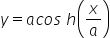at any point varies as

## Ordinate    Abscissa    Square of the abscissa    Square of the ordinateHint:

## The correct answer is: Square of the ordinate

### The given curve is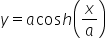Ordinate means the y-axisAnd abscissa means x-axisThe formula of length of the normal at point (x,y) is given by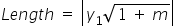Here, m =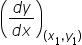Now, we will find the value of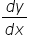We will substitute this value in the formula of length.Length is proportional to y12 as "a" is constant.We can see the length of the normal depends on the square of y. It means the length of normal varies square of ordinate.

For such questions, we should know the formula of length of normal.#### With Turito Foundation.#### Get an Expert Advice From Turito.